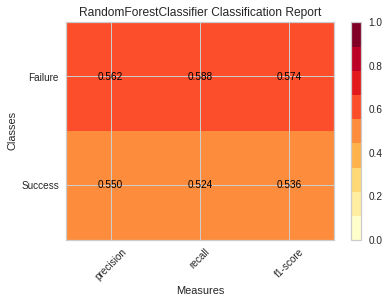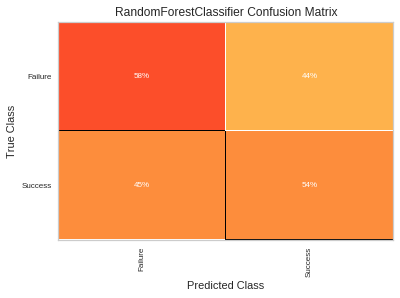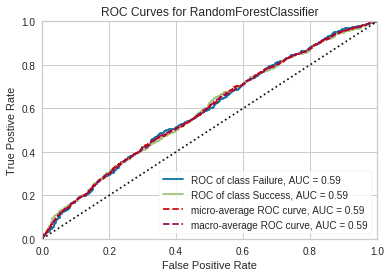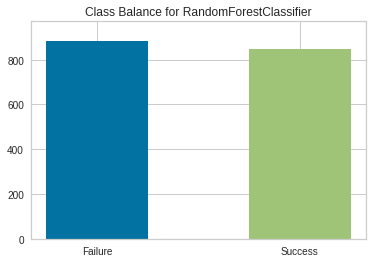## Machine Learning Model Visual Evaluation Example¶

In :
from sklearn.model_selection import train_test_split
from sklearn.externals import joblib
import pandas as pd
from yellowbrick.classifier import ClassificationReport, ConfusionMatrix, ROCAUC, ClassBalance
import numpy as np
import matplotlib
import matplotlib.pyplot as plt
%matplotlib inline

# Import previously created model see classify.ipynb
# Read in data then split data into training and test sets
# Create Label column defining whether or not the article's upvotes exceed the average vote for the subreddit
# Split data into training and test sets

pipeline = joblib.load('datascience.xz')
data = pd.read_csv('processed_datascience.csv.bz2')

data['z_scores'] = np.abs((data.ups-data.ups.mean())/data.ups.std())
data = data[data['z_scores']<= 2.5]

## Optional: Log transformation of up-votes
data['log_ups'] = np.log1p(data['ups'])

# Create Label column defining whether or not the article's upvotes exceed the average vote for the subreddit
data['gtavg'] = data.log_ups > data.log_ups.mean()

# Change Target label to Success or Failure
booldict = {True: 'Success', False: 'Failure'}
data['gtavg'] = data.gtavg.map(booldict)

train_X, test_X, train_y, test_y = train_test_split(data.title,
data.gtavg,
test_size=0.20,
random_state=25)


### Classification Report¶

In :
visualizer = ClassificationReport(pipeline)
visualizer.fit(train_X, train_y)
visualizer.score(test_X,test_y)
visualizer.poof()### Confusion Matrix¶

In :
cm = ConfusionMatrix(pipeline)
cm.fit(train_X, train_y)
cm.score(test_X, test_y)
cm.poof()### ROC AUC¶

In :
visualizer = ROCAUC(pipeline)
visualizer.fit(train_X, train_y)
visualizer.score(test_X, test_y)
g=visualizer.poof()### Class Balance¶

In :
visualizer = ClassBalance(pipeline)
visualizer.fit(train_X, train_y)
visualizer.score(test_X, test_y)
g=visualizer.poof()In [ ]: